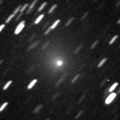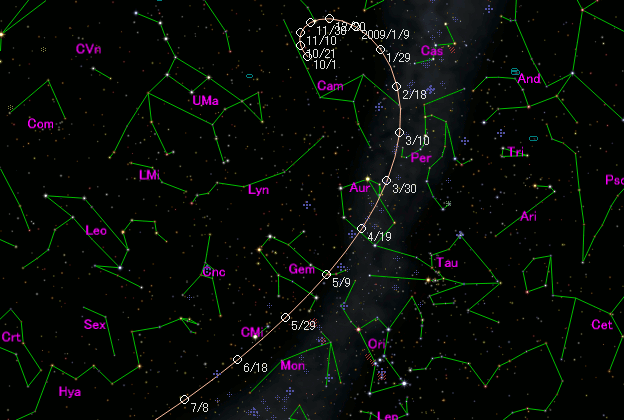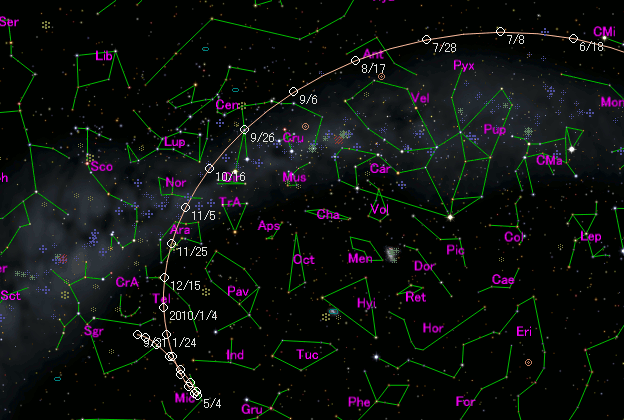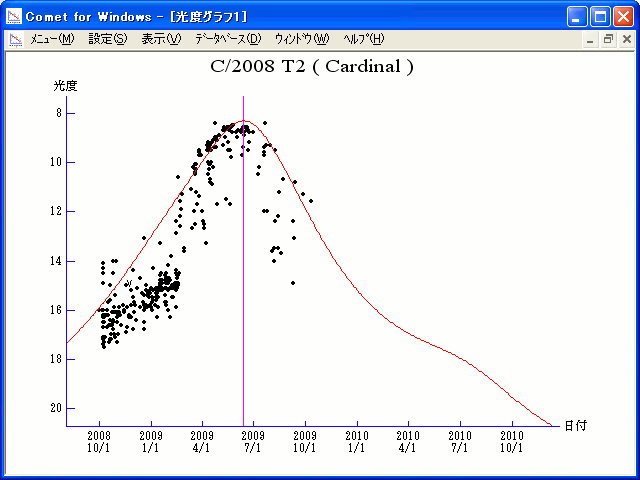# \$B%+!<%G%#%J%kWB@1(B

C/2008 T2 ( Cardinal )###\$B%W%m%U%#!<%k(B

 \$BH/8+F|(B 2008\$BG/(B10\$B7n(B1\$BF|(B \$BH/8+8wEY(B 16.0\$BEy(B \$BH/8+ Rob D. Cardinal (University of Calgary, Priddis)

###\$B###\$B50F;MWAG(B

```The following orbital elements are taken from MPC 66921:

Epoch 2009 June 18.0 TT = JDT 2455000.5
T 2009 June 13.2385 TT                                  MPC
q   1.202239             (2000.0)            P               Q
z  -0.000134       Peri.  215.8695      -0.7675781      +0.0280983
Node   309.6781      +0.5757120      -0.4089218
e   1.000161       Incl.   56.3041      -0.2817438      -0.9121367
From 1292 observations 2008 Oct. 6-2009 Aug. 14, mean residual 0".6.
Nongravitational parameters A1 = -1.35, A2 = +0.6263.
```

###\$B@1?^(B###\$B8wEYJQ2=(B

```        m1 = 6.0 + 5 log\$B&\$(B + 13.0 log r
```##### \$B50F;MWAG\$O(BMPC\$B\$N(BEphemerides and Orbital Elements\$B\$K7G:\\$5\$l\$?\$b\$N\$G\$9!#(B \$B@1?^\$O%9%F%i%J%S%2!<%?(B Ver.8 (\$B%"%9%H%m%"!<%D(B) \$B\$G:n@.\$7\$?\$b\$N\$G\$9!#(B \$B8wEY%0%i%U\$O(BComet for Windows\$B\$G:n@.\$7\$?\$b\$N\$G\$9!#(B